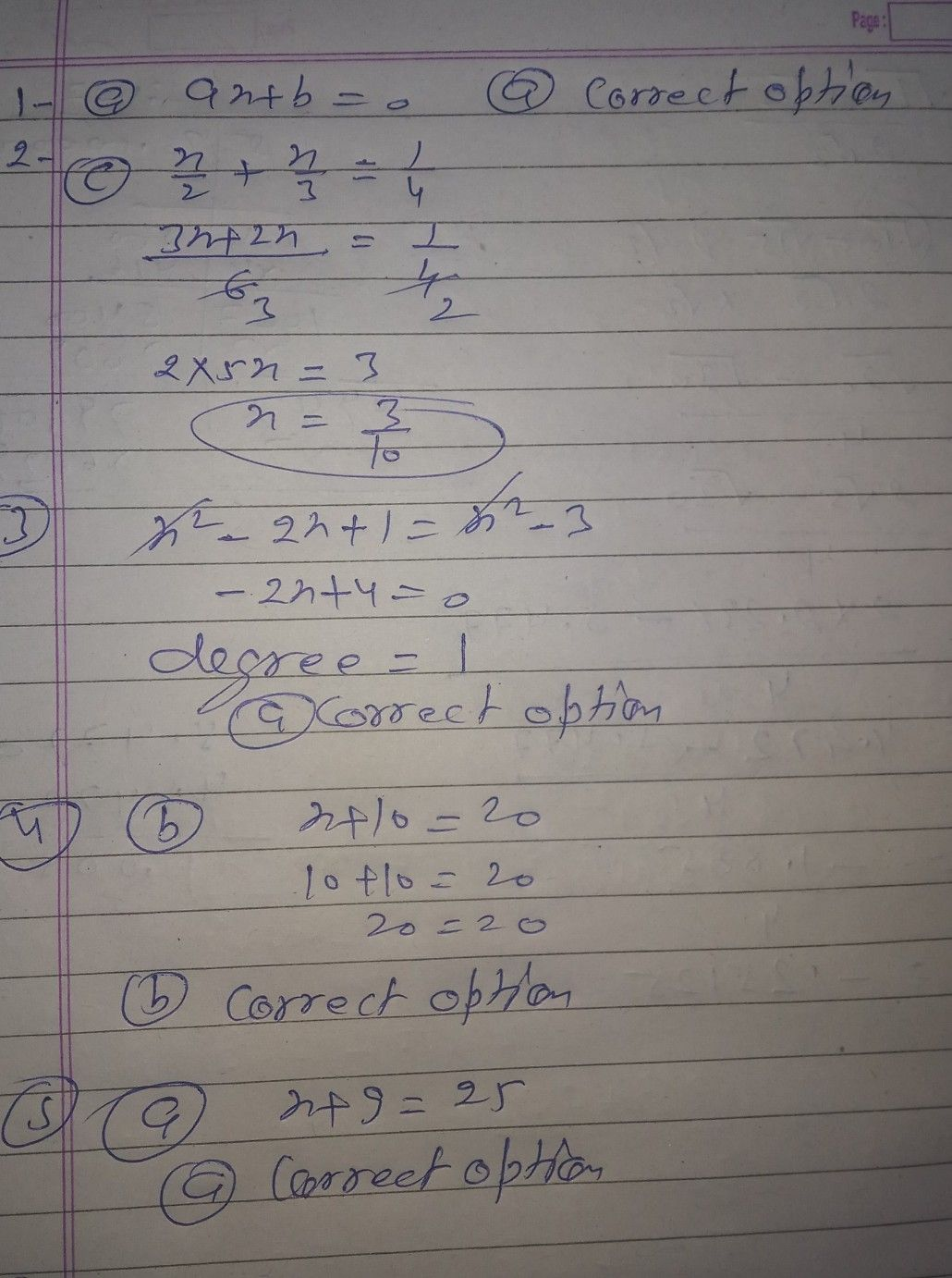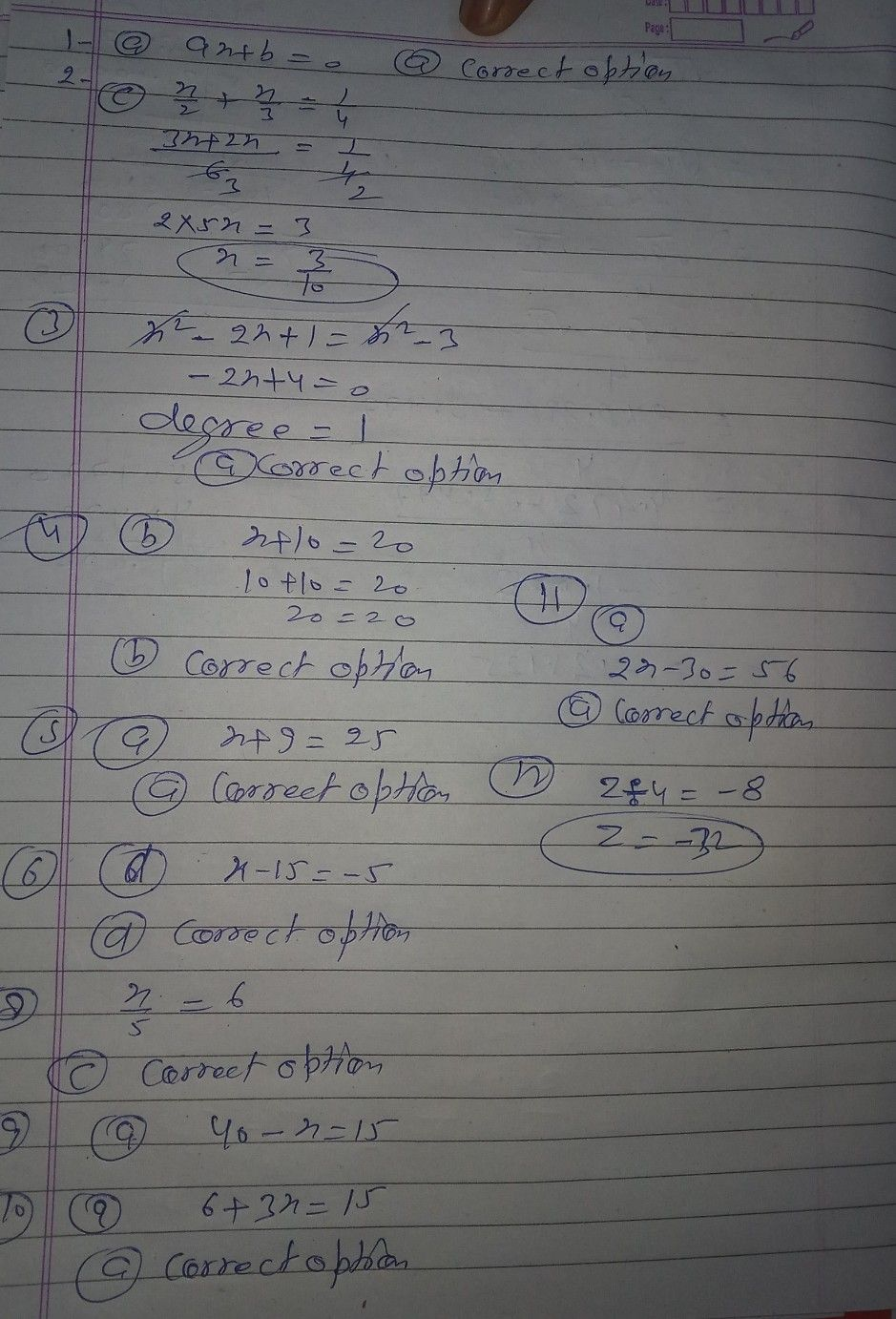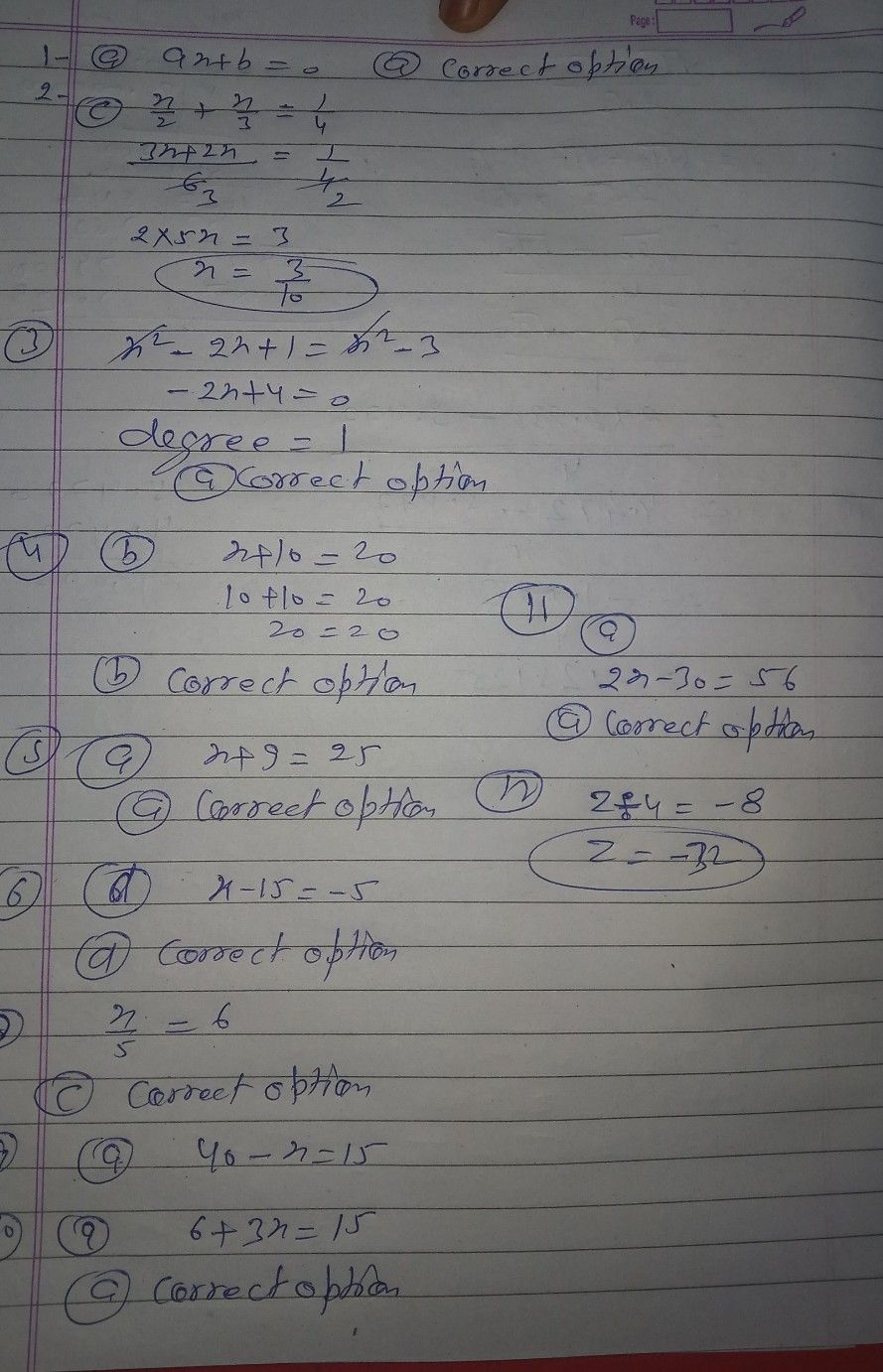Symbol
ProblemMultiple statement in the form of an equation is 1. The standard form of a linear equation 7. Seven times a number is 42. Thie (b) 7x 42 in one variable x is (a) x+ 7= 42 $\left(d\right)x-7=42$ (a) ax + b 0 (c) 7 = 42 (b) ax + bx + c = 0 (d) ax + bx + cx + dx +e = 0. 8. A number when divided by 5 gives 6. This statement in the form of an (c) ax+ bx + ex +d 0 Of the following, the linear equation in equation is 2. one variable x, is (a) x- 5 = 6 (b) $x+5=6$ x (b) -+ x-1 = 1 (d) 5x = 6. (0) = 6 (a) 4 4 (d) x + 2x +3 = 0. (c)+ 3 4 9. A number when subtracted from 40 3. The degree of the equation results into 15. This statement in the form of an equation is 2-2x + 1 x-3 is $x-40=15$ (a) 1 (b) 2 ((ac) ) $40-x=15$ $40+x=15$ ((b) d) $40x=15$ (c) 0 (d) 3. The statement 'on adding 10 in a 10. If 6 is added to 3 times of a number, it becomes 15. This statement in the form 4. number, the number becomes 20' in the of an equation is form of an equation is (a) x - 10 = 20 (b) $x+10=20$ (a) $3x+6=15$ (b) $3x-6=15$ (c) 10x 20 (d) 10 = 20. (c) $3x+15=6$ (d) $\dfrac {3x} {6}=15$ 5. If 9 is added to a number, it becomes 11. On subtracting 30 from two times a 25. This statement in the form of an number, we get 56. This statement in equation is the form of an equation is (a) x + 9 = 25 (b) x- 9 = 25 (a) $2x-30=56$ (b) $2x+30=56$ (c) 9x 25 (d) = 25. (c) $30-2x=56$ (d) $\dfrac {30} {2x}=56$ If 15 is subtracted from a number, it becomes- 5. This statement in the form 12. The root of the equation $z\div 4=-8is$ of an equation is (a) 3 (b) – 32 (a) $x+15=-5$ (b) $x-15=5$ (c) 12 (d) 4. (c) x+15 = 5 (d) $x-15=-5$
Algebra
SolutionQanda teacher - PREMLATAStudent
and so on...Qanda teacher - PREMLATA
okStudent